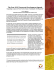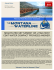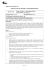# PROBLEM SET 6 FOR 18.102 DUE 7AM SATURDAY MARCH 28

## Transcription

PROBLEM SET 6 FOR 18.102 DUE 7AM SATURDAY MARCH 28
```PROBLEM SET 6 FOR 18.102
DUE 7AM SATURDAY MARCH 28, 2015.
Problem 6.1
Recall the space h2,1 (discussed in an earlier problem set) consisting of the complex valued sequences ci such that
X
kck2 =
(1 + |i|2 )|ci |2 < ∞.
i
Show that the unit ball in this space, considered as a subset of l2 , has compact
closure.
Problem 6.2
Define the space L2 (0, 1) as consisting of those elements of L2 (R) which vanish
outside (0, 1] and show that the quotient L2 (0, 1) = L2 (0, 1)/N (0, 1) by the null
functions in L2 (0, 1) is a Hilbert space.
Problem 6.3
Identify C[0, 1], the space of continuous functions on the closed interval, as a
subspace of L2 (0, 1). For each n ∈ N let Fn ⊂ L2 (0, 1) be the subspace of functions
which are constant on each interval ((m − 1)/n, m/n] for m = 1, . . . , n Show that
if f ∈ C[0, 1] there exists gn ∈ fn such that
δn =
sup
|f (t) − f (s)| =⇒ kf − gn kL2 ≤ δn .
|t−s|≤1/n
Problem 6.4
Show that a bounded and equicontinuous subset of C[0, 1] has compact closure in
L2 (0, 1). Note that equicontinuity means ‘uniform equicontinuity’ so for each δ > 0
there exists > 0 such that |x − y| < δ implies |f (x) − f (y)| < for all elements f
of the set.
Hint: Use the approximation by the finite dimensional spaces Fn .
Problem 6.5
If K ∈ C([0, 1] × [0, 1]) is a continuous function of two variables, show that
Z
(6.1)
Af (x) = K(x, y)f (y)
defines a compact linear operator on L2 (0, 1).
Hint: Show that A defines a bounded linear map from L2 (0, 1) to C[0, 1] and
that the image of the unit ball is equicontinuous using the uniform continuity of K.
Problem 6.6 – extra
Show that a closed and bounded subset of L2 (R) is compact if and only if it is
‘uniformly equicontinuous in the mean’ and ‘uniformly small at infinity’ so that for
1
2
PROBLEMS 6
each > 0 there exists δ > 0 such that
Z 1/δ
Z
|f |2 < 2 and |t| < δ =⇒ |f (x) − f (x − t)|2 < 2
−‘/δ
for all elements of the set.
Problem 6.7 – extra
Consider the space of continuous functions on R vanishing outside (0, 1) which
are of the form
Z x
v, v ∈ L2 (0, 1).
u(x) =
0
Show that these form a Hilbert space and that the unity ball of this space has
compact closure in L2 (0, 1).
```

### VX500 The compact 3D printer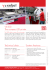### PDF Cut-Sheet - Wharfedale Pro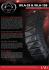### Spright Move Spright Compact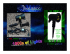### - SD Vidya Mandir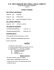### Lavandula angustifolia `Munstead Hishtil`S Strain`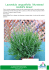### Flyer-AfIn_Training_190515_Accra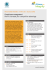### Hippie Dippie Tibbies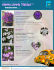### Saturday 22nd September 2012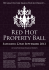### Ball Up Sports Flyer - Holland Junior School### Compact Panel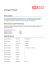### Throw a ball up and it will fall down 1) The force of gravity pulls the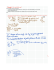### B2.63 - Dansk Energi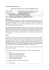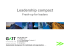### Ph.D. Qualifying Exam, Real Analysis Spring 2015, part I Do all five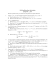### Compact Construction Equipment Market Analysis, Segments, Growth and Value Chain 2014 - 2020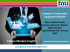Construction equipment industry comprises of a wide range of heavy machines which perform specific tasks for various applications. Construction equipment can be classified according to the size and capability of the construction machinery.

### Solutions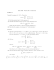### Fall 2015 Syllabus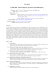### Tutorial Exercise (Week 6)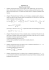### PROBLEM SET #7 SOLUTIONS AST142 1. Jeans Mass A spherical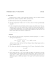### The Post-2015 Corporate Development Agenda